# ISEE Upper Level Quantitative : How to find the surface area of a sphere

## Example Questions

### Example Question #34 : Solid Geometry

In terms of, give the surface area, in square inches, of a spherical water tank with a diameter of 20 feet.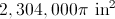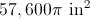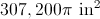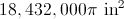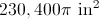Explanation:feet =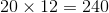inches, the diameter of the tank; half of this, or 120 inches, is the radius. Set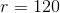, substitute in the surface area formula, and solve for: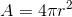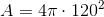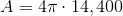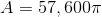### Example Question #9 : Spheres

Which is the greater quantity?

(a) The surface area of a sphere with radius 1

(b) 12

(a) and (b) are equal.

It is impossible to tell from the information given.

(b) is greater.

(a) is greater.

(a) is greater.

Explanation:

The surface area of a sphere can be found using the formula.

The surface area of the given sphere can be found by substituting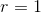: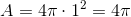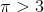so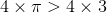, or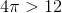This makes (a) greater.

### Example Question #1 : Spheres

Sphere A has volume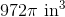. Sphere B has surface area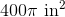. Which is the greater quantity?

(a) The radius of Sphere A

(b) The radius of Sphere B

(a) and (b) are equal

(a) is greater

It is impossible to tell from the information given

(b) is greater

(b) is greater

Explanation:

(a) Substitute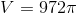in the formula for the volume of a sphere: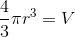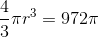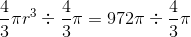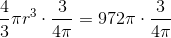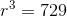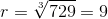inches

(b) Substitute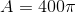in the formula for the surface area of a sphere: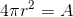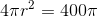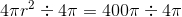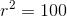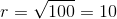inches

(b) is greater.

### Example Question #1 : How To Find The Surface Area Of A Sphereis a positive number. Which is the greater quantity?

(A) The surface area of a sphere with radius(B) The surface area of a cube with edges of length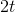It is impossible to determine which is greater from the information given

(A) and (B) are equal

(A) is greater

(B) is greater

The surface area of a sphere istimes the square of its radius, which here is; the surface area of the sphere in (A) is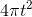.
The area of one face of a cube is the square of the length of an edge, which here is, so the area of one face of the cube in (B) is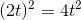. The cube has six faces so the total surface area is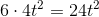.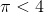, so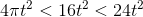, giving the sphere less surface area. (B) is greater.